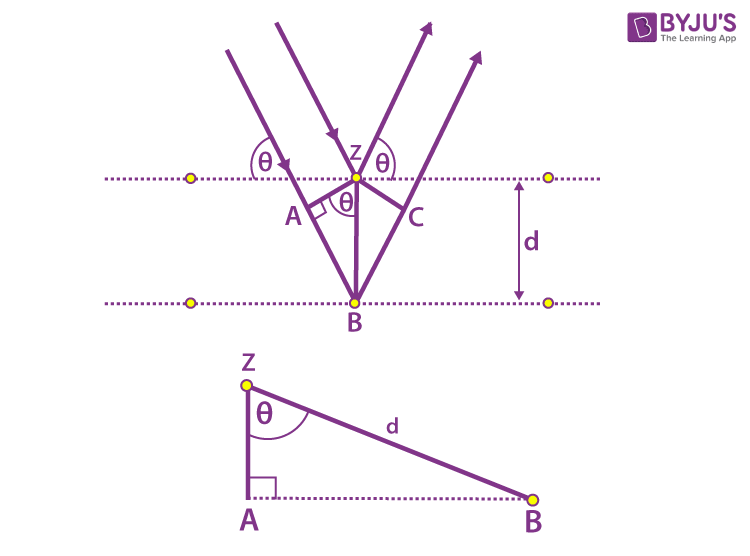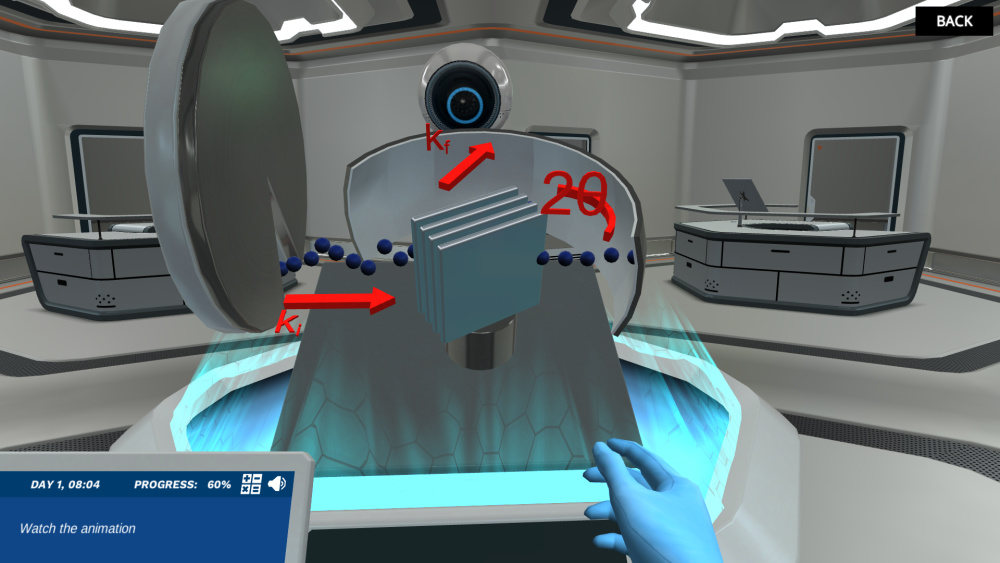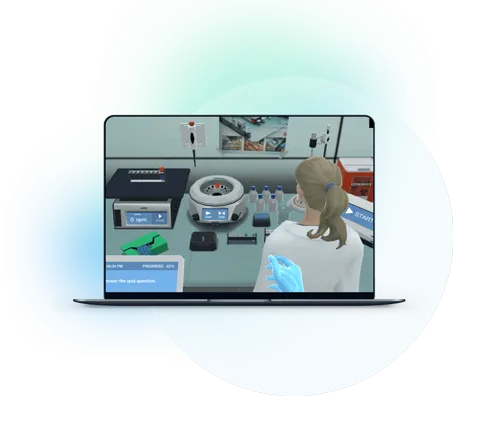Blog

# 5 Ways to Get Students Energized about Neutron Scattering: Bragg’s law

Teaching with Labster
October 11, 2022

Did you know that the colder it is, the faster your phone drains its battery? Is it possible to examine a battery's inner workings without damaging it? Yes, if you study its materials carefully.

But how’s it possible to study materials without disintegrating them?

Well, there is a technique to study the internal structure of substances known as Neutron Scattering.  All atoms' nuclei (except hydrogen) contain subatomic particles called neutrons. The neutrons in atoms can be released using a high-energy technique to create a free neutron beam that can pierce through nearly any substance.

Since neutrons have a wave nature, and the interplanar spaces of crystals are comparable to the wavelength of neutrons, they undergo diffraction.  When the beam of neutrons is diffracted from the atomic crystal lattice, we can determine its structure and size using Bragg’s law. The law defines the relationship between the wavelength, angle of incidence, and distance with interstitial spaces of atoms. So, the main application of this law is to study the internal structure of materials,

If you are teaching a quantum mechanics or advanced physics course, you may encounter this topic. However, teaching it may not be an easy task for you.

Read on to learn three reasons why Bragg’s law can be hard for students and further, we have listed five ways to make it more approachable for physics students.

## Why is Bragg’s law tricky for students?

Most Physics topics usually appeal to students due to their extensive real-life applications. However, students are not generally interested in abstract concepts like Bragg’s law. Here’s a list of three reasons why it is so.

### 1. Abstract Concept

When students hear out the word “law” in physics, they will usually think of it as a boring statement that they will have to memorize.

The same is the case here; the concept of Bragg’s law seems abstract to students since you can neither show them how the neutrons and x-rays are diffracted on the layers of microscopic atoms nor mention some daily-life applications. Though schematic diagrams to explain this process are available in textbooks, they don’t visually intrigue students.

### 2. Complex: Requires Grip of Mathematics

Bragg’s law is one of the complex laws of physics. Proper background knowledge of path difference, diffraction, coherence, and interference is required to understand this concept.

For the derivation of a mathematical expression, students need to know the basics of geometry and trigonometry. So, for students, the process is complex.

### 3. Lack of Practical Experimentation

In physics, you can understand most laws through practical experiments. However, in this case, you need neutrons for diffraction. To produce neutrons, you need a large-scale lab facility like a nuclear reactor or a particle accelerator with a metal target.

Setting up that kind of apparatus and experiment is not an option. Even, scientists who need to perform neutron-based experiments must travel to these science facilities for experimentation.

So, you are only left with one choice and that is the use of virtual lab simulations. We’ll discuss that part in the end.

## 5 Ways to make Bragg’s law an approachable topic for Students

Keeping in view the abstractness of this concept, we have listed five suggestions that will help you in making Bragg’s an approachable and interesting topic for your students.

### 1. Show Them People Behind Science

When teaching a dry topic like Bragg’s law, an effective way to grab students’ attention is through stories. You can tell them the story of the people behind that theory or how they created it. Moreover, the tales of scientists help them remember the law in the longer term.

The Father and Son who Proposed Bragg’s law

The famous Bragg’s law we study today was actually studied by a young son, Lawrence Bragg, and his father, William Henry Bragg. At that time, X-ray diffraction was being studied by another German scientist, Laue. He proposed that X-rays are waves and undergo diffraction. However, Lawrence’s father thought of X-rays as streams of particles. The nature of X-rays was still unknown at that time.

In 1912, Lawrence Bragg while strolling by the river had an epiphany that crystals constitute parallel sheets of atoms. If the X-rays struck at the top layer of their surface, diffraction won’t take place since the collision would be out of phase, canceling out each other. However, if the rays strike the space between layers, then they would be in phase, producing a diffraction pattern on nearby sheets. Based on his realization, he proposed a theory that defined the relationship between the wavelength of X-rays, incident angle, and the interstitial space between atoms of crystals.

Later, to test the law, his father constructed a mechanism that allowed a crystal to be rotated over a range of angles and the reflected energy was measured. With this setup, they were able to determine the exact spacing of the atomic sheets in several different types of simple crystals.

Moreover, they used the equation to determine the wavelengths of X-rays produced by various metallic targets, they calculated atomic spacing using the weight of the crystal and the Avogadro constant. This helped them understand the nature of X-rays from metallic targets.

The simple Bragg’s equation also led them to study the internal crystal structures of NaCl, diamond, and ZnS. For that, they were awarded a Nobel prize in 1915. Lawrence Bragg was just 25 when he received the Nobel prize, making him the youngest recipient.

In the later years, when neutron was discovered, neutron diffraction was used to study the intricate structure of materials. Neutron diffraction is an excellent alternative to X-ray diffraction since neutrons are more penetrating and they can provide deeper visuals.

### 2. Give the Overview of Basic Terms

As we mentioned earlier, to understand Bragg’s law, students need to know the basic concepts associated with it. So, whenever you introduce this law to students, make sure you start with the basic terms.

The first concept to explain in this context is diffraction. Diffraction is the bending of waves around the ends of an obstacle. However, not all obstacles create diffraction; it only occurs when the size of the obstacle is comparable to the wavelength of the beam/wave.

Diffraction is the property associated with waves only. As neutrons have a wave-particle nature they undergo neutron diffraction when hit on an atomic structure since their wavelength is comparable to interatomic spaces. Bragg’s law which was initially proposed based on X-ray diffraction also applies to neutron scattering.

Another important term is Interference. The phenomenon of interference occurs when two coherent waves of the same frequency meet at a point. They can either interfere constructively or destructively based on their path difference. Constructive interference means waves reinforce and their amplitudes are added while destructive means waves cancel out each other.

Path difference at a point is the difference in distance traveled by two waves at the intersecting point. If the path difference is an integral multiple of lambda (), constructive interference occurs, while if the path difference is an odd multiple of λ/2 , destructive interference occurs.

### 3. Explain the Mathematical Relation

For some students, Physics topics become interesting when you justify things from a Mathematical perspective. Moreover, if students know how the final relation of Bragg’s law was established, it becomes easier for them to remember and memorize it for their exams.

You can formulate the mathematical expression using this simple schematic diagram. Consider two beams of X-ray incident on a crystal structure. One beam hits point Z, and it reflects and travels upwards. The same happens to the second beam at point B.Schematic Diagram of X-ray diffraction (Bragg’s Law) (Image Source

The path difference between the two waves is the extra distance traveled by the second beam.

Path Difference = AB + BC

Also from the figure,  AB = BC, So:

Path Difference = AB + AB=2AB  ---(1)

If we separate the right-triangle ABZ, the value of AB can be written in terms of layer spacing “d”. AB is the vertical component of “d”.

AB = dsin---(2)

Substitute the value of AB in equation (1).

Path Difference=2 dsin

For constructive interference, the path difference must be an integral multiple of lambda i.e. nλ. So, the final equation becomes:

nλ=2 dsin

This is known the Bragg’s equation or bragg’s law.

### 4. Relate it to the Real World: Show Its Applications

Bragg’s law seems to be abstract and you normally don’t find its daily-life applications. However, Bragg’s equation has been extremely helpful to chemists in determining the structure of molecules, and sub-atomic structures such as ions, protons, etc.

X-rays and neutrons both obey Bragg’s law and can be used to determine the structure of materials. However, since neutrons don’t interact with matter, they can easily penetrate into heavy objects like combustion engines. So, for studying the internal working of an engine or any complex structure, neutron scattering is extremely helpful. One can study the internals just without dissembling the structure, just as human X-rays give you a picture of your internal body.

### 5. Use Labster’s Virtual Lab Simulations

Neutron scattering experiment obviously cannot be performed since it requires a controlled environment or large scientific facilities to produce electrons. However, with computer simulations, students can easily study the technique.

In this regard, Labster provides an opportunity to teachers to engage through interactive learning scenarios. Labster virtual labs simulate experiments and train them with lab techniques through a 3D visual experience.You can watch the process of animation neutron diffraction in our Neutron Scattering: Investigating Bragg's Law Virtual Lab. In this simulation, students will check out the internal layers of a battery using neutron scattering and study how temperature affects the scientific equipment.What exactly are virtual labs?